Next: WEIGHTS AND CONSTRAINTS Up: Data modeling by least Previous: Data modeling by least

# MORE EQUATIONS THAN UNKNOWNS

When there are more linear equations than unknowns, it is usually impossible to find a solution which satisfies all the equations. Then one often looks for a solution which approximately satisfies all the equations. Let a and c be known and x be unknown in the following set of equations where there are more equations than unknowns.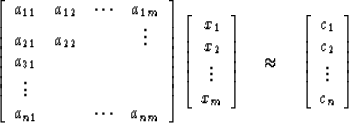(1)

Usually there will be no set of xi which exactly satisfies (1). Let us define an error vector ej by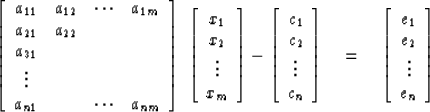(2)
It simplifies the development to rewrite this equation as follows (a trick I learned from John P. Berg).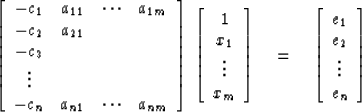(3)
We may abbreviate this equation as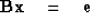(4)
where B is the matrix containing c and a. The ith error may be written as a dot product and either vector may be written as the column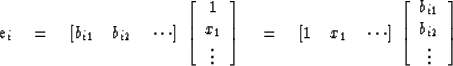Now we will minimize the sum squared error E defined as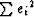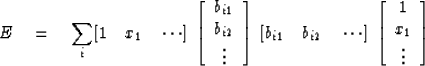(5)
The summation may be brought inside the constants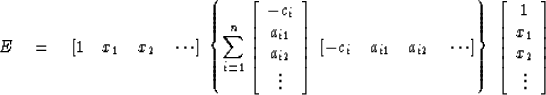(6)
The matrix in the center, call it rij, is symmetrical. It is a positive (more strictly, nonnegative) definite matrix because you will never be able to find an x for which E is negative, since E is a sum of squared ei. We find the x with minimum E by requiring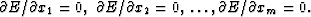Notice that this will give us exactly one equation for each unknown. In order to clarify the presentation we will specialize (6) to two unknowns.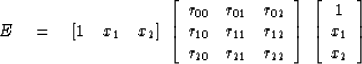(7)
Setting to zero the derivative with respect to x1, we get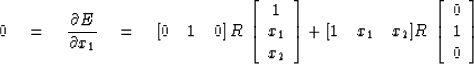(8)
Since rij = rji, both terms on the right are equal. Thus (8) may be written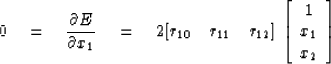(9)
Likewise, differentiating with respect to x2 gives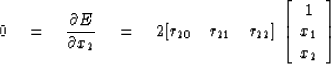(10)
Equations (9) and (10) may be combined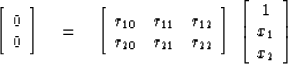(11)
This form is two equations in two unknowns. One might write it in the more conventional form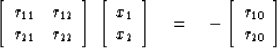(12)
The matrix of (11) lacks only a top row to be equal to the matrix of (7). To give it that row, we may augment (11) by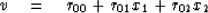(13)
where (13) may be regarded as a definition of a new variable v. Putting (13) on top of (11) we get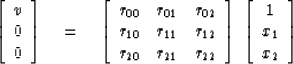(14)
The solution x of (12) or (14) is that set of xk for which E is a minimum. To get an interpretation of v, we may multiply both sides by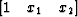, getting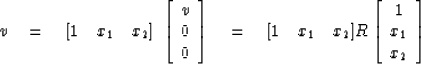(15)

Comparing (15) with (7), we see that v is the minimum value of E.

Occasionally, it is more convenient to have the essential equations in partitioned matrix form. In partitioned matrix form, we have for the error (6)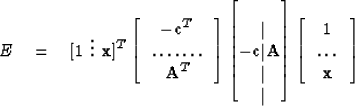(16)
The final equation (14) splits into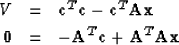(17) (18)
where (18) represents simultaneous equations to be solved for x. Equation (18) is what you have to set up in a computer. It is easily remembered by a quick and dirty (very dirty) derivation. That is, we began with the overdetermined equations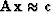;premultiplying by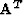gives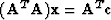which is (18).

In physical science applications, the variable zj is frequently a complex variable, say zj = xj + iyj. It is always possible to go through the foregoing analyses, treating the problem as though xi and yi were real independent variables. There is a considerable gain in simplicity and a saving in computational effort by treating zj as a single complex variable. The error E may be regarded as a function of either xj and yj or zj and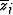. In general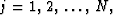but we will treat the case N = 1 here and leave the general case for the Exercises. The minimum is found where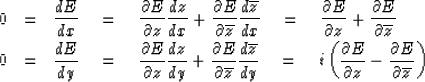(19) (20)
Multiplying (20) by i and adding and subtracting these equations, we may express the minimum condition more simply as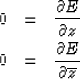(21) (22)

However, the usual case is that E is a positive real quadratic function of z and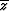and that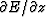is merely the complex conjugate of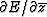. Then the two conditions (21) and (22) may be replaced by either one of them. Usually, when working with complex variables we are minimizing a positive quadratic form like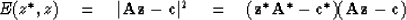(23)
where * denotes complex-conjugate transpose. Now (22) gives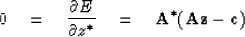(24)
which is just the complex form of (18).

Let us consider an example. Suppose a set of wave arrival times ti is measured at sensors located on the x axis at points xi. Suppose the wavefront is to be fitted to a parabola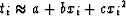.Here, the xi are knowns and a, b, and c are unknowns. For each sensor i we have an equation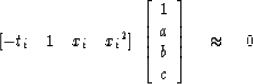(25)
When i has greater range than 3 we have more equations than unknowns. In this example, (14) takes the form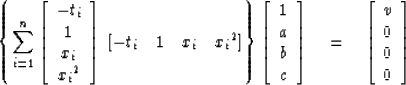(26)
This may be solved by standard methods for a, b, and c.

The last three rows of (26) may be written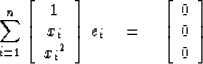(27)
This says the error vector ei is perpendicular (or normal) to the functions 1, x, and x2, which we are fitting to the data. For that reason these equations are often called normal equations.

## EXERCISES:

1. Extend (24) by fitting waves observed in the x, y plane to a two-dimensional quadratic.
2. Let y(t) constitute a complex-valued function at successive integer values of t. Fit y(t) to a least-squares straight line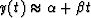where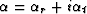and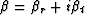. Do it two ways: (a) Assume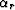,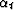,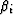, and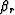are four independent variables, and (b) Assume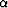,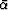,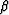, and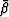are independent variables. (Leave answer in terms of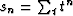.)
3. Equation (14) has assumed all quantities are real. Generalize equation (14) to all complex quantities. Verify that the matrix is Hermitian.
4. At the jth seismic observatory (latitude xj, longitude yj) earthquake waves are observed to arrive at time tj. It has been conjectured that the earthquake has an origin time t, latitude x, and longitude y. The theoretical travel time may be looked up in a travel time table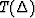where T is the travel time and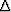is the great circle angle. One has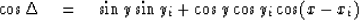The time residual at the jth station, supposing that the earthquake occurred at (x, y, t), is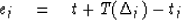The time residual, supposing that the earthquake occurred at (x + dx, y + dy, t + dt), is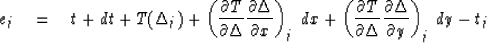Find equations to solve for dx, dy, and dt which minimize the sum-squared time residuals.
5. Gravity gj has been measured at N irregularly spaced points on the surface of the earth (colatitude xj, longitude yj, j = 1, N). Show that the matrix of the normal equation which fits the data to spherical harmonics may be written as a sum of a column times its transpose, as in the preceding problem. How would the matrix simplify if there were infinitely many uniformly spaced data points? (NOTE: Spherical harmonics S are the class of functions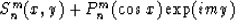for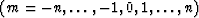and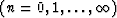where Pmn is an associated Legendre polynomial of degree n and order m.)
6. Ocean tides fit sinusoidal functions of known frequencies quite accurately. Associated with the tide is an earth tilt. A complex time series may be made from the north-south tilt plus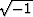times the east-west tilt. The observed complex time series may be fitted to an analytical form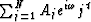. Find a set of equations which may be solved for the Aj which gives the best fit of the formula to the data. Show that some elements of the normal equation matrix are sums which may be summed analytically.
7. The general solution to Laplace's equation in cylindrical coordinates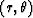for a potential field P which vanishes at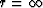is given by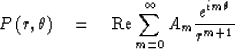Find the potential field surrounding a square object at the origin which is at unit potential. Do this by finding N of the coefficients Am by minimizing the squared difference between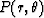and unity integrated around the square. Give the answer in terms of an inverse matrix of integrals. Which coefficients Am vanish exactly by symmetry?Next: WEIGHTS AND CONSTRAINTS Up: Data modeling by least Previous: Data modeling by least
Stanford Exploration Project
10/30/1997